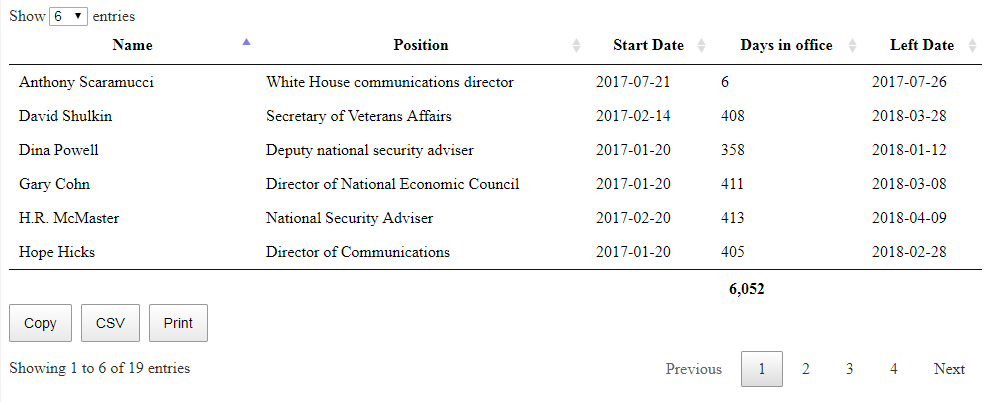# more fun with datatables

I actually don’t get a chance to do much GUI development but I did get a short stint on a project that used datatables.  It is actually fascinating just how much of a facelift this does give your standard HTML.  I did a small example of this in my blog entry software development the easy way.

Between normal javascript and datatables it is possible to actually make web applications that have a feel of your standard windows heavy client application.

The only example I have to share today is the ability to do a bit more with datatables.  In this case the need was to add totals of the contents of the table as a summary line.  This isn’t so difficult if you already know what the total is but if you do not there is a small bit of code that will do the calculation for you.

```         function intVal ( i ) {
return typeof i === 'string' ?
i.replace(/[\\$,]/g, '')*1 :
typeof i === 'number' ?
i : 0;
};

\$('#example').DataTable( {
"footerCallback": function ( row, data, start, end, display ) {
var api = this.api(), data;

var ageTotal = api
.column( 3 )
.data()
.reduce( function (a, b) {
return intVal(a) + intVal(b);
}, 0 );

ageTotal = Number(ageTotal).toFixed(2);
ageTotal = ageTotal.toLocaleString();

\$( api.column( 3 ).footer() ).html(Number(ageTotal).toLocaleString());
},
```

If you add this code, actually not much different than many other examples on the internet to your web site then all of a sudden you will see your footer change with the new value(s).The code is not all that difficult to follow.  You can use this particular function to put any values that you wish into the table footer.  In my case it was a simple sum, but if you needed some fancy algorithm then this would be the place to put it.